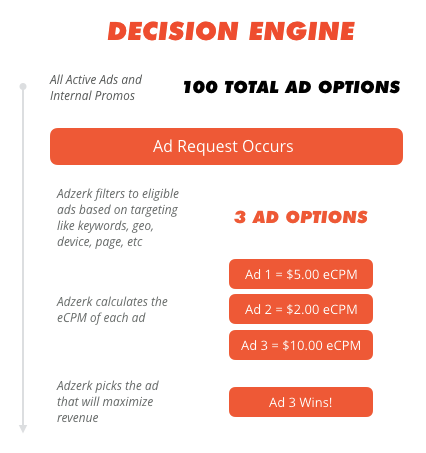# Overview

Auctions are a delivery method with the goal of generating the most revenue for each ad impression. Effectively, rather than picking an ad randomly via a lottery system, it calculates the expected revenue (eCPM) you'll make for each ad and selects the ad that'll deliver the highest return.

It's used when:

• You have multiple advertisers with different bids
• You've set the expectation that bid amount will impact volume (versus guaranteeing a certain number of impressions/clicks)
• You are charging on CPC or CPA (versus straight CPM or flat fee)
• Your inventory is competing against RTB inventory# What Is eCPM?

Kevel uses effective Cost-Per-Mille (thousand impressions), or eCPM, to calculate the value of each ad in auction. It's the historical revenue of an ad per 1000 impressions:

``````eCPM = Price per Event * (Number of Revenue generating events / Number of Impressions) * 1000

For example, for CPM:
CPM = \$5 (for 1,000 impressions)
Price per event  = \$5 / 1,000 = \$0.005
Number of Revenue generating events (impressions in this case) = Number of Impressions = 9000

So, by plugging the values into the formula above, you can see that eCPM = CPM

eCPM = \$0.005 * (9,000 / 9,000) * 1,000
= \$0.005 * 1 * 1,000
= \$0.005 * 1,000
eCPM = \$5 = CPM
``````

If your flights use a CPM rate, the eCPM is the same as CPM because each impression generates the same amount of revenue. However, if you are charging on a cost-per-click (CPC) or cost-per-action (CPA) basis, revenue is not derived from impressions, so eCPM has to be calculated using click-through-rate and/or conversion-rate:

``````We use the same calculation as above to calculate eCPM for CPC and CPA:

eCPM = Price per Event * (Number of Revenue generating events / Number of Impressions) * 1000

For example, for CPC/CPA:
NOTE: we don't have to divide the CPC/CPA by 1,000, because the price is already per event:
CPC/CPA = Price per event (click or another action) = \$5
Number of Revenue generating events (clicks or another action) = 18
Number of Impressions = 9000

So, by plugging the values into the formula above, you can calculate the eCPM

eCPM = \$5 * (18 / 9000) * 1000
= \$5 * 0.002 * 1000
= \$5 * 2
eCPM = \$10

Note that this portion of the formula:
Number of Revenue generating events / Number of Impressions
is essentially the click-through-rate (CTR)
So, in the example above our CTR:
CTR = 18 / 9000
= 0.002
CTR = 0.2%
``````

Comparing eCPMs to select the winner of the auction is useful for the following reasons:

• It enables CPC and CPA flights to compete against each other and against CPM flights
• It allows CPC and CPA flights that generate the most revenue to win the auction (instead of competing using their price).

Just because an advertiser is paying a higher CPC doesn't mean they'll generate the most revenue. In the scenario below, the \$1.00 CPC bidder actually makes 150% more than the \$2.00 bidder because their click-through-rate (CTR) is so much higher:

CPC

Clicks

CTR (per 1000 impressions)

Revenue

eCPM

\$1.00

50

5%

\$50

\$50

\$1.50

20

2%

\$30

\$30

\$2.00

10

1%

\$20

\$20

### 🚧

Flat rate flights can also compete in auctions, but their eCPMs cannot be calculated. Instead, a fixed eCPM must be used.

# How Kevel Calculates eCPM For Auctions

If a flight has eCPM Optimization settings enabled, Kevel will calculate an eCPM for the ads in the flight approximately every thirty minutes. (You can define the historical time period for the optimization and the default eCPM for this calculation using the eCPM Optimization settings).

To calculate the eCPM, we use the:

• Total revenue from the time period specified for the calculation
• The number of impressions the ad has served during the time period

We then multiply this eCPM by the multiplier value (if applicable).

If the calculated eCPM exceeds the max eCPM from the flight settings, will we use the max eCPM as the final eCPM for the auction. (Also, if the calculated eCPM is lower than the min CPM, we will use that instead.) Otherwise, we will use the calculated eCPM.

If a flight is still in burn-in mode, we will use the default eCPM specified on the flight.Updated 3 months ago

### What's Next

 Second Price Auctions Setting Up an Auction

# Auctions / eCPM Optimization

### Suggested Edits are limited on API Reference Pages

You can only suggest edits to Markdown body content, but not to the API spec.